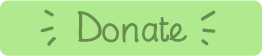# Aztec Take Away (start at 50, then race to 0)

### Learning Objectives:

• Year 1: Read, write and interpret mathematical statements involving addition (+), subtraction (−) and equals (=) signs.
• Year 1: Represent and use number bonds and related subtraction facts within 20
• Year 1: Add and subtract one-digit and two-digit numbers to 20, including 0
• Year 2: Recall and use addition and subtraction facts to 20 fluently, and derive and use related facts up to 100
• Year 2: Add and subtract numbers using concrete objects, pictorial representations, and mentally, including: a two-digit number and 1s,a two-digit number and 10s...
• Year 3: add and subtract numbers mentally, including: a three-digit number and 1s, a three-digit number and 10s, a three-digit number and 100s.

### Instructions:

1. Split your class into two teams (red & green).
2. Red team's player is on the left. Their pot of goo is on the right, above the other team's head!
3. The winner is the first team to reach zero, (and pour their coloured goo on the other team's head!)
5. The subtractions are between 1 and 12. There is no 0 in this new version of the game.
6. Don't forget to talk about how you worked it out!
7. Press 'check'.
8. Correct answers will rotate the pot.
9. Answer incorrectly and you miss a turn.

Have fun!Made using theandlibraries.